# 資料科學在量化金融中的應用：指數預測（下）## 特徵工程

• 處理缺失值並提取所需變數
• 資料標準化
• 處理分類變數

### 1.  處理缺失值並提取所需變數

``````x_input = (df_model.dropna()[['Year','Month','Day','Weekday','seasonality','sign_t_1','t_1_PricePctDelta','t_2_PricePctDelta','t_1_VolumeDelta']].reset_index(drop=True))``y = df_model.dropna().reset_index(drop=True)['AdjPricePctDelta']``

### 2.  資料標準化

``````scaler = StandardScaler()
x = x_input.copy()
x[['t_1_PricePctDelta','t_2_PricePctDelta','t_1_VolumeDelta']]=scaler.fit_transform(x[['t_1_PricePctDelta','t_2_PricePctDelta','t_1_VolumeDelta']])``````

### 3.  處理分類變數

``````x_mod = pd.get_dummies(data=x, columns=['Year','Month','Day','Weekday','seasonality'])
x_mod.columns``````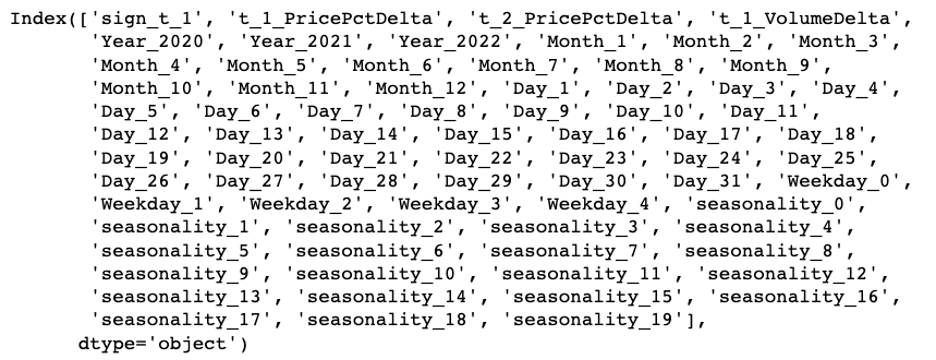``x_mod.shape``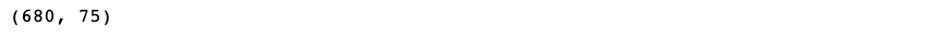## 模型評估

``trail_result = ensemble_method_reg_trails(x_train, y_train, x_test, y_test)``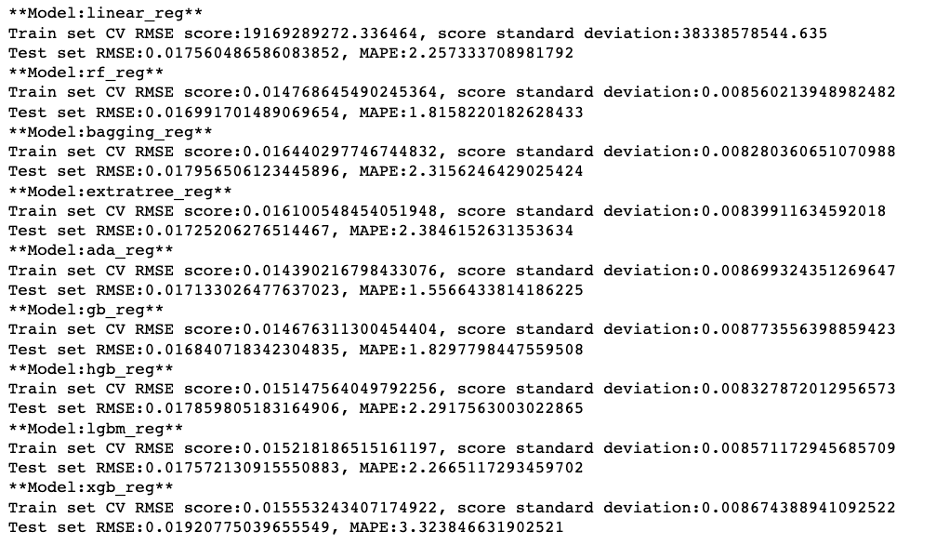``pd.DataFrame(trail_result).sort_values('model_test_mape', ascending=True)``• 使用 RandomizedSearchCV 尋找最佳引數的大致範圍
• 使用 GridSearchCV 尋找更精確的引數

• n_estimators
• base_estimator
• learning_rate

base_estimator 是 ada boost 提升演算法的基礎，我們需要提前建立一個 base_estimator 的列表。

``````l_base_estimator = []
for i in range(1,16):
base = DecisionTreeRegressor(max_depth=i, random_state=42)
l_base_estimator.append(base)
l_base_estimator += [LinearSVR(random_state=42,epsilon=0.01,C=100)]``````

### 1.  使用 RandomizedSearchCV 尋找最佳引數的大致範圍

``````randomized_search_grid = {'n_estimators':[10, 50, 100, 500, 1000, 5000],
'base_estimator':l_base_estimator,
'learning_rate':np.linspace(0.01,1)}``````
``````search = RandomizedSearchCV(AdaBoostRegressor(random_state=42),
randomized_search_grid,
n_iter=500,
scoring='neg_mean_absolute_error',
n_jobs=-1,
cv=5,
random_state=42)
result = search.fit(x_train, y_train)``````

``result.best_params_````result.best_score_``### 2.  使用 GridSearchCV 尋找更精確的引數

• n_estimators: 1-50
• base_estimator: Decision Tree with max depth 9
• learning_rate: 0.7左右
``````search_grid = {'n_estimators':range(1,51),
'learning_rate':np.linspace(0.6,0.8,num=20)}``````GridSearchCV 的結果如下：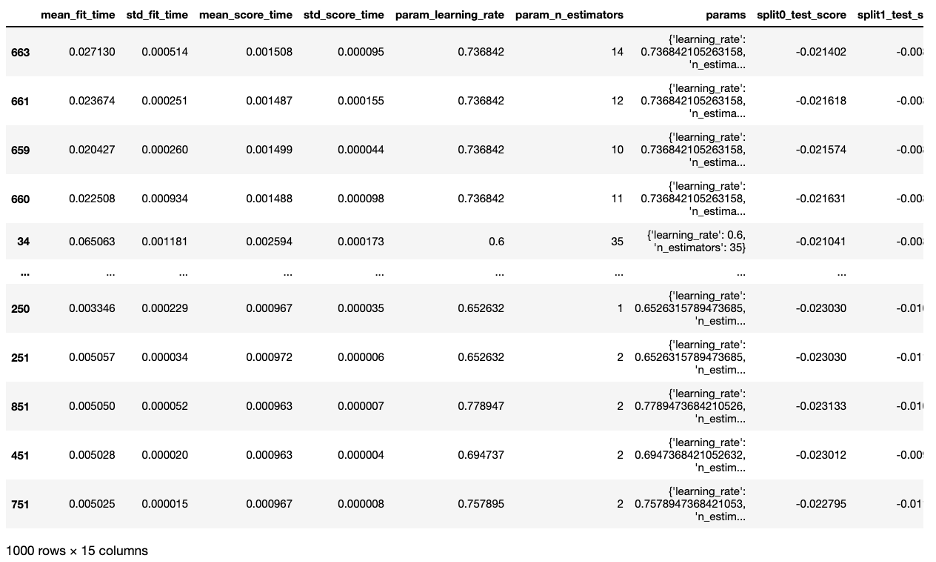``best_reg.fit(x_mod, y)````````m_forecast = best_reg.predict(x_mod)
mean_absolute_percentage_error(y, m_forecast)``````## 模型預測

``````def forecast_one_period(price_info_adj_data, ml_model, data_processor):
# Source data: Data acquired straight from source
next_day = last_record['Date'] + relativedelta(days=1)
next_day_t_2_PricePctDelta = last_record['t_1_PricePctDelta']
next_day_t_1_VolumeDelta = last_record['Volume_in_M'] - last_record['t_1_VolumeDelta']
if next_day_t_1_PricePctDelta > 0:
next_day_sign_t_1 = 1
else:
next_day_sign_t_1 = 0
# Value -99999 is a placeholder which won't be used in the following modeling process
next_day_input = (pd.DataFrame({'Date':[next_day],
'Volume_in_M':[-99999],
't_1_PricePctDelta':[next_day_t_1_PricePctDelta],
't_2_PricePctDelta':[next_day_t_2_PricePctDelta],
't-1volume': last_record['Volume_in_M'],
't-2volume': last_record['t-1volume'],
't_1_VolumeDelta':[next_day_t_1_VolumeDelta],
'sign_t_1':next_day_sign_t_1}).set_index('Date'))
# If forecast period is post Feb 15, 2020, input data starts from 2020-02-16,
# as our model is dedicated for market under Covid Impact.
# Another model could be used for pre-Covid market forecast.
if next_day > datetime.datetime(2020, 2, 15):
# Add new record to original data for modeling preparation
# Prep for modeling
x,y = data_processor.data_modeling_prep(input_modified)
next_day_x = x.iloc[-1:]
forecast_price_delta = ml_model.predict(next_day_x)
# Consolidate prediction results
forecast_df = {'Date':[next_day], 'price_pct_delta':[forecast_price_delta], 'actual_pct_delta':[np.nan]}
return pd.DataFrame(forecast_df)``````## 分析預測結果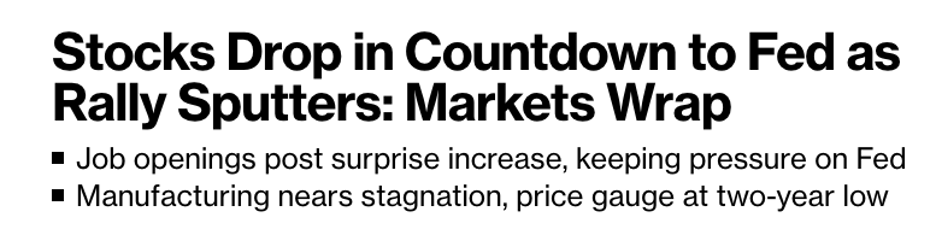“Stocks finished lower as data showing a solid US labor market bolstered speculation that Federal Reserve policy could remain aggressively tight even with the threat of a recession.”

## 總結

• 確定預測目標：反映北美股票市場的指數 — 標普500 ；
• 資料收集：從公共金融網站下載歷史價格資料；
• 探索性資料分析：初步瞭解資料的特性，資料視覺化，將時間序列資訊以影象的形式呈現；
• 資料預處理：將時間轉換為變數，更改價格資料，尋找週期和季節性，根據週期調整交易量資料；
• 資料工程：處理缺失值並提取所需變數，資料標準化，處理分類變數；
• 模型選擇和訓練：拆分訓練集和測試集，確定模型方向和評估指標，嘗試訓練各種模型；
• 模型評估：根據指標選定最優模型，使用 RandomizedSearchCV 尋找最佳引數的大致範圍，再使用 GridSearchCV 尋找更精確的引數；
• 模型預測：整合輸入資料，預測未來一個工作日的價格變動；
• 分析預測結果：結合當日的實際情況，理解市場變動，發揮模型價值。